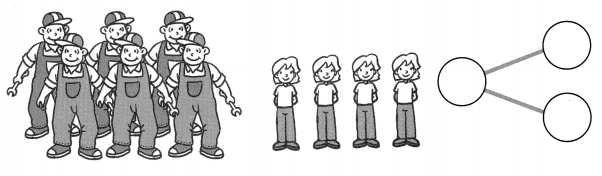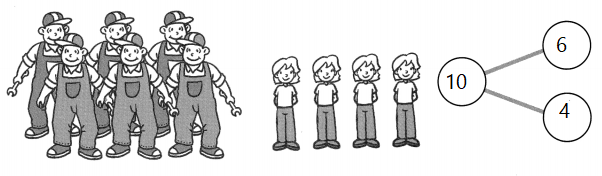# Math in Focus Grade 1 Chapter 2 Practice 2 Answer Key Making Number Bonds

This handy Math in Focus Grade 1 Workbook Answer Key Chapter 2 Practice 2 Making Number Bonds detailed solutions for the textbook questions.

## Math in Focus Grade 1 Chapter 2 Practice 2 Answer Key Making Number Bonds

Look at the pictures.

Complete the number bonds.

Example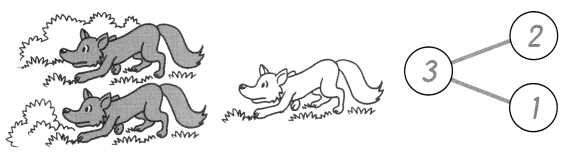Question 1.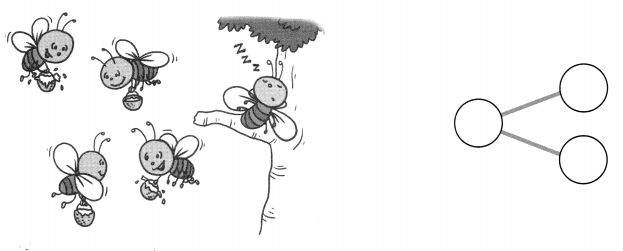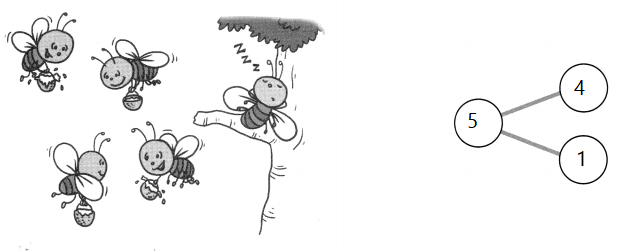Explanation:
4 + 1 = 5
so, total there are 5 bees

Question 2.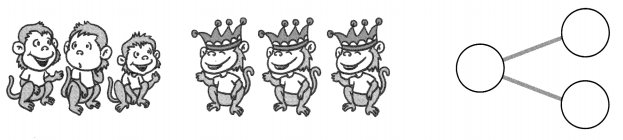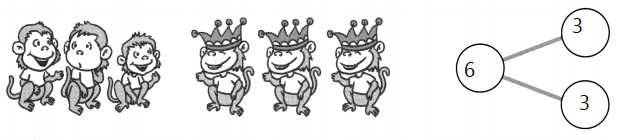Explanation:
Three monkeys are with crowns and three monkeys are with out crowns
so, 3 + 3 = 6
The sum of 3 and 3 is 6

Look at the pictures. Complete the number bonds.

Question 3.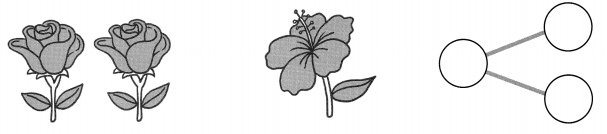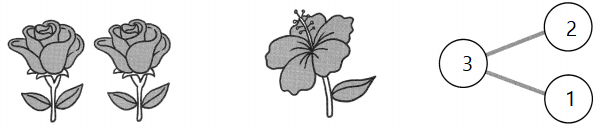Explanation:
2 + 1 = 3
There are 2 roses and a hibiscus
The sum of two and one is 3

Question 4.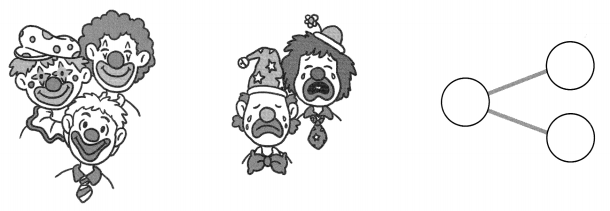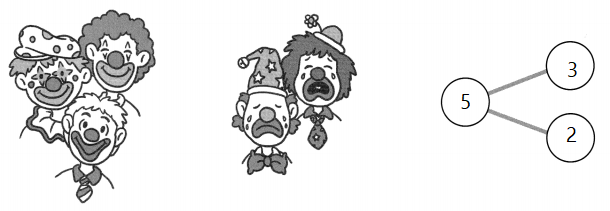Explanation:
There are 5 jokers
Three are smiling jokers and 2 are crying jokers
3 + 2 = 5
The sum of 3 and 2 is 5

Question 5.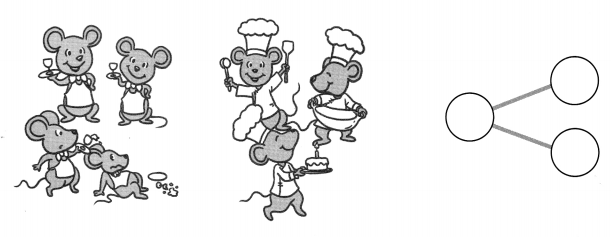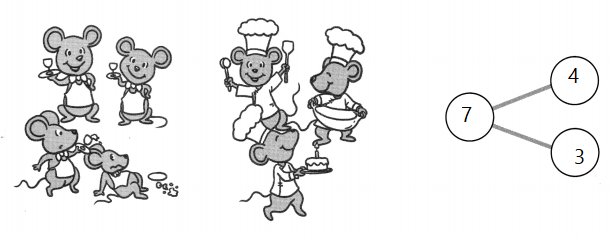Explanation:
Four mice are waiting in the restaurant  and three mice are preparing the food
3 + 4 = 7
the sum of 4 and 3 is 7

Question 6.